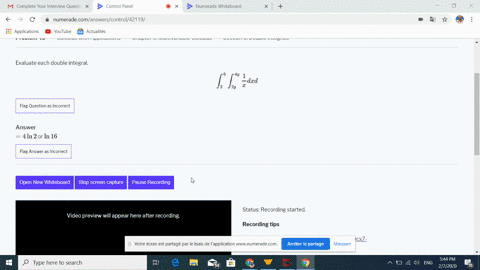Black Friday is Here! Start Your Numerade Subscription for 50% Off!Join Today## Discussion

You must be signed in to discuss.

## Video Transcript

Okay, so here we have the stubble Intergroup Zira one four and then zero to you X of squaring X was why? Why the ex so first has taken anti derivative with respect to why it's straightforward. So this is just next bus y to the three halves divided by three house. But that's the same thing is multiplying by two thirds Elvis. Spectre that out. And then we're evaluating this from zero to x. And when we get to thirds Roland for replaying X, we get, uh, two X to the three house minus zero. We get X to the three halves and okay, so this is two to the three halves, minus times. Exit three has planets one. So what we can do here is actually factor out toe stagger out next to the three halves, which would give us a constant of two to the three halves minus one. And they were just have one for X to the three. Have Jax. Okay, so you have two thirds and then two to the three halves. Nice one. This is going to be X to the five has two by the bed. Five has evaluated from one before Okay. Good. And so this will be in the being four. Over fifteen. Then we have two to the three halves. This one and then exited. Five house. Evaluate from one to four. Well, this should be thirty two. Four to the five houses. Thirty to one already. No, it's good.